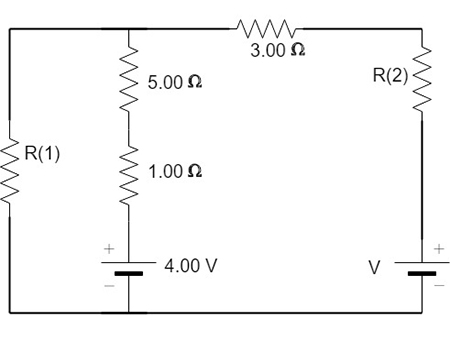# The circuit shown in the figure below is connected for 2.90 min. (Assume R1 = 6.20 Ohms, R2 =...

## Question:

The circuit shown in the figure below is connected for 2.90 min. (Assume R1 = 6.20 {eq}\Omega {/eq}, R2 = 1.40 {eq}\Omega {/eq}, and V = 18.0 V.) (a) Determine the current in each branch of the circuit. (b) Find the energy delivered by each battery. (c) Find the energy delivered to each resistor. (d) Identify the type of energy storage transformation that occurs in the operation of the circuit. (e) Find the total amount of energy transformed into internal energy in the resistors.## Kirchoff's law:

Kirchhoff?s junction law states that the algebraic sum of the currents into any branch point is zero. If the currents entering the branch point are taken as positive and currents leaving as negative, the algebraic sum of currents into a branch point must be zero.

Applying Kirchoff's law

{eq}V = R_2I+3I+5I_1+I_1+4 {/eq}

{eq}18-4 = 1.4I+3I+5I_1+I_1 {/eq}

{eq}14 = 4.4 I+6I_1 ----1 {/eq}

Now applying...

Become a Study.com member to unlock this answer! Create your account